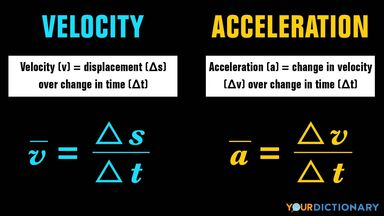# Difference Between Velocity and Acceleration Explained

You may know that both velocity and acceleration have something to do with how fast an object moves. You’re right, but what’s the difference between these two terms in physics? Keep reading for more information about velocity, acceleration, and how to tell them apart.difference between velocity and acceleration

## The Main Difference Between Velocity and Acceleration

Velocity and acceleration both use speed as a starting point in their measurements. Speed, which is the measurement of distance traveled over a period of time, is a scalar quantity. Both velocity and acceleration are vector quantities, meaning that they use both magnitudes and a specified direction.

Here are the basic definitions of velocity and acceleration:

• velocity - the rate of displacement of a moving object over time
• acceleration - the rate of velocity change over time

As you can see, velocity requires speed for its measurement, and acceleration requires velocity for its measurement. In order to properly measure any of these quantities, you’ll need to know how both velocity and acceleration work.

## The Function of Velocity

The difference between an object’s starting point and its ending location is its amount of displacement. Two possible values of velocity are an object’s average velocity (the average calculation of its velocity over one time period) and its instantaneous velocity (its velocity in one moment in time). You can measure velocity in the following units:

• m/s - meters per second (used by The International System of Units and Measurements)
• kph - kilometers per hour (used by countries that use the metric system)
• mph - miles per hour (used in the United States)

If an object’s velocity is lower than its speed, you can tell that it did not travel in one direction. You need to measure an object’s velocity before you can record any increases in its speed — also known as acceleration.

## The Function of Acceleration

Pressing your foot to the gas pedal makes your car accelerate. But what does that mean in physics? When an object’s velocity and acceleration point in the same direction, your speed increases. If its velocity and acceleration point in different directions, your speed decreases, or decelerates.

Like velocity, acceleration can be measured by an object’s average acceleration (the average amount of acceleration over a period of time) or its instantaneous acceleration (its acceleration rate in one instant). Constant acceleration indicates that an object’s acceleration rate was constant in its motion. Acceleration can be constant when the force acting on an object is gravity.

## Velocity and Acceleration Equations

Finding an object’s average velocity means that you know its displacement, or change in position (Δs), the change in time during its journey (Δt), and the direction traveled. The equation for average velocity (v) looks like this:

v = Δs/Δt

It gets a bit more complicated when calculating an object’s average acceleration (a). For that, you’ll need to know its change in velocity (Δv) and the change in time (Δt). It looks like this:

a = Δv/Δt

Basically, you are figuring out the rate of change in meters per second squared (m/s^2). This solution will tell you the proper acceleration for an object over a set period of time.

## Velocity, Acceleration, and Speed

Understanding the relationship between velocity and acceleration can help you predict where an object will go given certain variables. It’s just one way for us to learn about how the laws of physics affect nearly everything we do. If you’d like to learn more about the concept of speed, check out an article that discusses the main difference between speed and velocity.